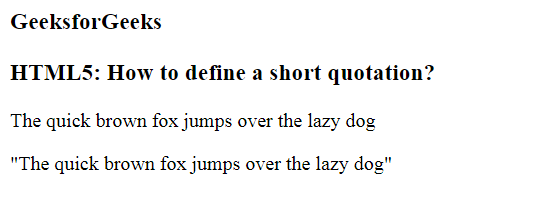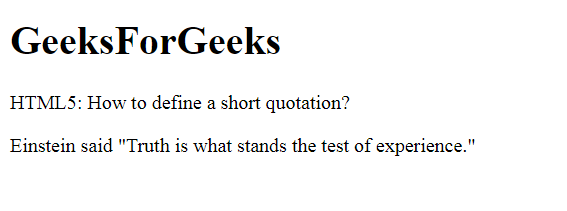# How to Add a Short Quotation using HTML5 ?

In this article, we will define a short quotation by using an <q> tag. It is used to insert quotation texts on a web page, i.e. a portion of texts different from the normal texts.

Syntax:

`<q> Quotation Text... </q>`

Example 1:

 ` ` `<``html``> ` ` `  `<``head``> ` `    ``<``title``> ` `        ``Define a short quotation ` `    `` ` ` ` ` `  `<``body``> ` `    ``<``h3``>GeeksforGeeks ` ` `  `    ``<``h3``> ` `        ``HTML5: How to define  ` `        ``a short quotation? ` `    `` ` ` `  `    ``<``p``> ` `        ``The quick brown fox jumps  ` `        ``over the lazy dog ` `    `` ` ` `  `    ``` `    ``<``p``><``q``> ` `        ``The quick brown fox jumps  ` `        ``over the lazy dog ` `    `` ` ` ` ` `  ` `

Output:Example 2:

 ` ` `<``html``> ` ` `  `<``head``> ` `    ``<``meta` `charset``=``"utf-8"``> ` `    ``<``title``> ` `        ``How to define a  ` `        ``short quotation ` `    `` ` ` ` ` `  `<``body``> ` `    ``<``h1``> ` `        ``GeeksForGeeks ` `    `` ` ` `  `    ``<``h2``> ` `        ``Define a short quotation? ` `    `` ` ` `  `    ``<``p``> ` `        ``Einstein said <``q``>Truth is what ` `        ``stands the test of experience. ` `    `` ` ` ` ` `  ` `

Output:Supported Browsers: